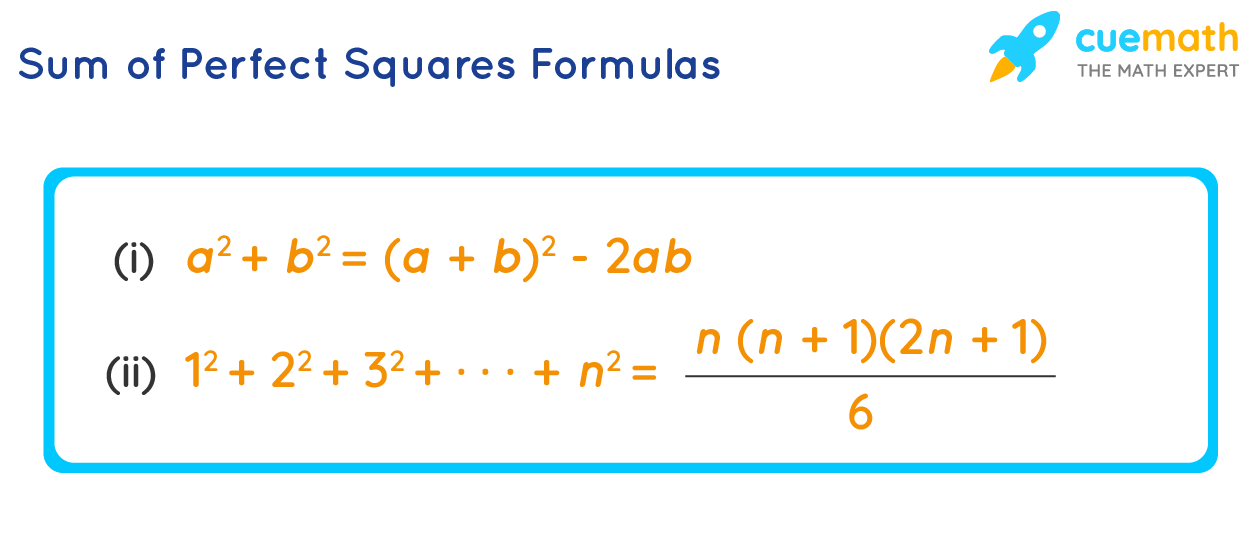# Sum of Perfect Squares Formula

Before knowing what is the sum of the perfect squares formula, first, let us recall what are perfect squares. A perfect square is a number that can be written as the square of a number. 1, 4, 9, 16, 25, 36, etc are some perfect squares as they can be expressed as 12, 22, 32, 42, 52, 62, etc respectively. The sum of perfect squares formula is used to find the sum of two or more perfect squares without adding them manually.

## What Is the Sum of Perfect Squares Formula?

We have two types of formulas for finding the sum of perfect squares. One is the formula to find the sum of two perfect squares, the other formula is to find the sum of the first "n" perfect squares.

• ### The formula for finding the sum of the squares for first "n" natural numbers is:12 + 22 + 32 + ... + n2 = [ n (n + 1) (2n + 6) ] / 6Let us see the applications of the sum of perfect squares formulas in the following section.

## Solved Examples Using Sum of Perfect Squares Formula

### Example 1: Find the sum of squares of 101 and 99.

Solution:

To find: The sum of squares of 101 and 99. i.e., 1012 + 992.

Using the sum of perfect squares formula:

a2 + b2 = (a + b)2 - 2ab

Substitute a = 101 and b = 99 in the above formula:

1012 + 992 = (101 + 99)2 - 2(101)(99)​​​

= (200)2 - 2 (9999)

= 40000 - 19998

= 20,002

Answer: 1012 + 99= 20,002.

### Example 2: Find the sum of squares of the first 25 natural numbers.

Solution:

To find: The sum of the first 25 natural numbers.

Substitute n = 25 in the sum of the perfect squares formula of the first n natural numbers:

12 + 22 + 32 + ... + n2 = [ n (n + 1) (2n + 6) ] / 6

12 + 22 + 32 + ... + 252 = [ 25 (25 + 1) (2(25) + 1) ] / 6

= ( 25 × 26 × 51 ) / 6

= 5,525

Answer: The sum of the first 25 natural numbers = 5,525.